# SAT II Math II : Mode

## Example Questions

### Example Question #1 : Mode

Find the mode of the following data set: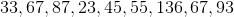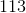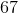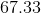Explanation:

Find the mode of the following data set:The mode will be the most repeated number. To find it, simply see which number appears most.

In this case, we have two 67's, and only 1 of each other term.

Therefore, our mode is 67.

### Example Question #2 : Mode

Determine the mode of the following numbers: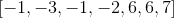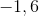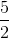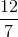Explanation:

The mode is defined as the number or numbers that has the highest frequency in the data set.

The numbersappear in the data set twice, while the other numbers appear once.

This means that the mode is:### Example Question #3 : Mode

Calculate the mode: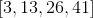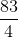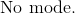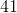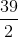Explanation:

The definition of mode is the number or numbers that have the highest frequency in a data set.

There is only one of each number given in the data set.

This means that there is no mode.

The answer is:### Example Question #4 : Mode

Determine the mode: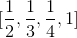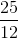Explanation:

The mode includes all numbers that have more than one frequency.  Since every number only occurs once in the set of data, there is no mode.

The answer is:### Example Question #5 : Mode

Determine the mode of the numbers: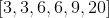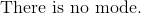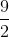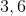The answer is: